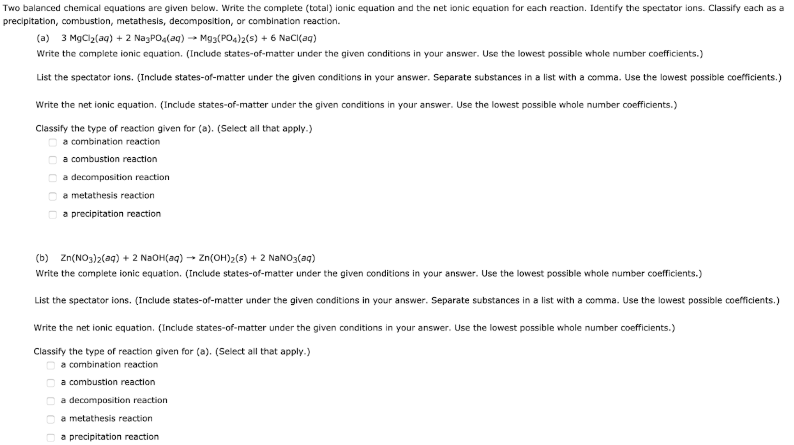# Two balanced chemical equations are given below. Write the complete (total) ionic equation for each reaction. Identify the spectator ions. Classify each as a precipitation, combustion, metathesis, decomposition, or combination reaction. (a) 3 MgCl2(aq) + 2 Na3PO4(aq) -> Mg3(PO4)2(s) + 6 NaCl(aq) Write the complete ionic equation. (Include states-of-matter under the given conditions in your answer. Use the lowest possible whole number coefficients.) List the spectator ions. (Include states-of-matter under the given conditions in your answer. Separate substances ina list with a comma. Use the lowest possible whole number coefficients.) Write the net ionic equation. (Include states-of-matter under the given conditions in your answer. Use the lowest possible whole number coefficients.) Classify the type of reaction given for (a). (Select all that apply.) a. combination reaction b. a combustion reaction c. a decomposition reaction d. a metathesis reaction e. a precipitation reaction (b) Zn(NO3)2(aq) + 2 NaOH(aq) -> Zn(OH)2(s) + 2 NaNO3(aq) Write the complete ionic equation. (Include states-of-matter under the given conditions in your answer. Use the lowest possible whole number coefficients.) List the spectator ions. (Include states-of-matter under the given conditions in your answer. Separate substances ina list with a comma. Use the lowest possible whole number coefficients.) Write the net ionic equation. (Include states-of-matter under the given conditions in your answer. Use the lowest possible whole number coefficients.) Classify the type of reaction given for (a). (Select all that apply.) a. combination reaction b. a combustion reaction c. a decomposition reaction d. a metathesis reaction e. a precipitation reaction